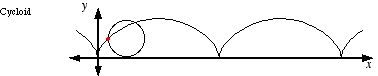index: click on a letter A B C D E F G H I J K L M N O P Q R S T U V W X Y Z A to Z index index: subject areas numbers & symbols sets, logic, proofs geometry algebra trigonometry advanced algebra & pre-calculus calculus advanced topics probability & statistics real world applications multimedia entrieswww.mathwords.com about mathwords website feedback

 Cycloid The path traced by a point on a wheel as the wheel rolls, without slipping, along a flat surface. The standard parametrization is x = a(t – sin t), y = a(1 – cos t), where a is the radius of the wheel. Note: Cycloids are periodic functions.See also